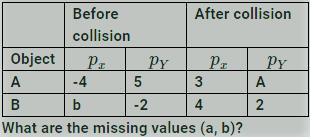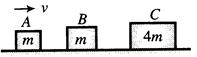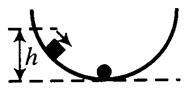Courses

# QUIZ 1:centre Of Mass,linear Momentum(#Free Test Series)

## 15 Questions MCQ Test Physics chapterwise notes and mocks JEE | QUIZ 1:centre Of Mass,linear Momentum(#Free Test Series)

Description
This mock test of QUIZ 1:centre Of Mass,linear Momentum(#Free Test Series) for JEE helps you for every JEE entrance exam. This contains 15 Multiple Choice Questions for JEE QUIZ 1:centre Of Mass,linear Momentum(#Free Test Series) (mcq) to study with solutions a complete question bank. The solved questions answers in this QUIZ 1:centre Of Mass,linear Momentum(#Free Test Series) quiz give you a good mix of easy questions and tough questions. JEE students definitely take this QUIZ 1:centre Of Mass,linear Momentum(#Free Test Series) exercise for a better result in the exam. You can find other QUIZ 1:centre Of Mass,linear Momentum(#Free Test Series) extra questions, long questions & short questions for JEE on EduRev as well by searching above.
QUESTION: 1

Solution:
QUESTION: 2

Solution:
QUESTION: 3

### Three particles of masses 1 kg, 2 kg and 3 kg are situated at the comers of an equilateral triangle move at speed 6ms-1, 3ms-1 and 2ms-1 respectively. Each particle  maintains a direction towards the particle at the next corner symmetrically. Find velocity of CM of the system at this 2kg instant

Solution:
QUESTION: 4

Two particles A and B start moving due to their mutual interaction only. If at any timet, aand aB are their respective accelerations, vand vB are their respective velocities, and up to that time Wand Ware the work done on A and B respectively by the mutual force, mand mB are their masses respectively, then which of the following is always correct?

Solution:
QUESTION: 5

The centre of mass of a non-uniform rod of length L whose mass per unit length λ varies as λ=kx2/Lwhere k is a constant and x is the distance of any point on rod from its one end, is (from the same end)

Solution:
QUESTION: 6

In a system of particles 8 kg mass is subjected to a force of 16 N along +ve x−axis and another 8 kg mass is subjected to a force of 8 N along +ve y−axis. The magnitude of acceleration of centre of mass and the angle made by it with x−axis are given respectively by

Solution:
QUESTION: 7

In a gravity free space, a man of mass M standing at a height h above the floor, throws a ball of mass m straight down with a speed u. When the ball reaches the floor, the distance of the man above the floor will be

Solution:
QUESTION: 8

The velocities of two particles A and B of same mass are VA=aî and VB=bj^ where a and b are constants. The acceleration of particle A is (2ai^+4bj^) and acceleration of particle B is (ai^−4bj^) (in m/s2). The centre of mass of two particle will move in

Solution:
QUESTION: 9

A bar of mass ′m′ length ℓ is pure translator motion with its centre velocity v. It collides with another identical bar which is in rest and sticks to it. Assume that after the collision it becomes one system, then the angular velocity of the system after the collision is

Solution:
QUESTION: 10

An object of mass 3m splits into three equal fragments. Two fragments have velocities vj^ and vi^. The velocity of the third fragment is

Solution:
QUESTION: 11

Distance of the centre of mass of a solid uniform cone from its vertex is z0. If the radius of its base is R and its height is h then z0 is equal to

Solution:
QUESTION: 12

Two objects that are moving along an xy-plane on a frictionless floor collide. Assume that they form a closed, isolated system. The following table gives some of the momentum components (in kilogram meters per second) before and after the collision. Before collision After collisionSolution:
QUESTION: 13

A ball is projected in a direction inclined to the vertical and bounces on a smooth horizontal plane. The range of one rebound is R. If the coefficient of restitution is e, then range of the next rebound is

Solution:
QUESTION: 14

Three blocks are initially placed as shown in the figure. Block A has mass m and initial velocity v to the right. Block B with mass and block C with mass 4m are both initially at rest. Neglect friction. All collisions are elastic. The final velocity of blocks A isSolution:
QUESTION: 15

A block of mass m starts from rest and slides down a frictionless semi-circular track from a height h as shown. When it reaches the lowest point of the track, it collides with a stationary piece of putty. also having mass m. If the block and the putty stick together and continue to slide, the maximum height that the block-putty system could reach isSolution: